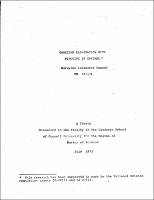## Gaussian Elimination with Pivoting is Optimal##### Abstract
V. Strassen discovered that two matrices of order 2 could be multiplied using 7 multiplications and 18 additions of numberss and has shown that two matrices of order n could be multiplied in less than $4 \cdot 7 n^{\log_{2}7}$ operations, an operation being defined as a multiplication, division, subtraction or addition. Also he has shown that the classical Gaussian elimination is not optimal by giving an algorithm to compute the inverse of a nonsingular matrix with certain principal submatrices nonsingular in less than $5 \cdot 64 n^{\log_{2}7}$ operations. In other words, Strassen's algorithm provides no room for pivoting. J. Bunch and J. Hopcroft have got rid of the above anomaly and have shown how to obtain the triangular factorization of a permutation of a nonsingular matrix in less than $2 \cdot 44 n^{\log_{2}7}$ operations and the inverse in less than$6 \cdot 83 n^{\log_{2}7}$ operations. In this thesis it is shown, using the results of Strassen and Bunch and Hopcroft, that Gaussian elimination with pivoting is optimal in the sense that the bound for the number of operations required to do Gaussian elimination is the least for "sufficiently large" systems of equations. Also expressions are derived for the various coefficients in the bounds for the various procedures that arise in solving linear systems of equations with the general assumption that two matrices of order u could be multiplied in p multiplications and q additions of numbers.
1973-07
##### Publisher
Cornell University
##### Keywords
computer science; technical report
##### Previously Published As
http://techreports.library.cornell.edu:8081/Dienst/UI/1.0/Display/cul.cs/TR73-176
technical report